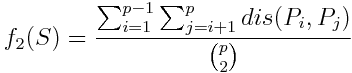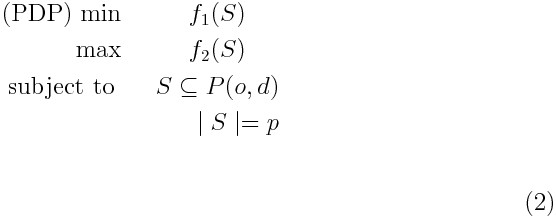Path Dissimilarity Problem

Martí, Campos, Resende and Duarte (2012)
University of Valencia (Spain), AT&T Labs (USA) and University Rey Juan Carlos (Spain)

Problem Description

The path dissimilarity problem (PDP) (see Dell'Olmo, Gentili and Scozzari, 2005) is a bi-objective routing problem in which a set of p paths from an origin to a destination must be generated with minimum length and maximum dissimilarity. Finding different paths in a graph is a classical optimization problem. The best known is the

s-shortest path problem in which the shortest, second shortest, s-th shortest paths from an origin o to a destination d are obtained in a graph. However, many of these alternative paths are likely to share a large number of edges. This is why in some applications we need to consider an alternative approach. For example, in the context of hazmat transportation we want to obtain spatially dissimilar paths that minimize the risk (distributing the risk over all regional zones to be crossed uniformly).

Given an undirected graph G=(V, E) with V the set of vertices and E the set of edges with associated cost cij for (i, j) in E, and a pair of origin-destination vertices, o-d, we define P(o, d) as the set of all paths in G from o to d. Note that in most applications the cost cij of edge (i, j) is its Euclidean distance. Given an integer number p > 1 , a feasible solution to the path dissimilarity problem, PDP, is a set S \subseteq P(o, d) such that|S| = p. Given a solution S={P1, P2,…, Pp}, we define its value f1(S) as the average of the costs of the paths in S:We also define its dissimilarity value f2(S) as the average of the dissimilarity between the distinct pairs of paths in S:As applied in Martí, González-Velarde and Duarte A. (2009) the dissimilarity dis(Pi,Pj) between two paths Pi and Pj is computed as the average of the distances between each vertex in Pi to the path Pj plus the average of the distances between each vertex in Pj to the path Pi.

With these elements, we can formulate the PDP as:State of the Art Methods

The most relevant procedures developed to solve this problem are:

• ITS: Iterative Penalty Method. Johnson et al. (1992)
• GTS: Gateway Shortest Path. Lombard and Chrch (1993)
• MM: Minimax method. Kuby et al. (1997)
• IPM_pD: IPM for p-dispersion problem. Akgun e al. (2000)
• MSPA: Multicriteria Shortest Path Algorithm. Dell'Olmo et al. (2005)
• GRASP: Greedy ramdomized adaptive search procedure. Martí et al (2009)
• GRASP+PR: Multiobjective GRASP with Path Relinking. Martí et al (2012)

Instances (PDP)

We have compiled a comprehensive set of benchmark problems including all problem instances which have so far been used for conducting computational experiments for the \pdp. The test problems are taken from the well known TSP Library and are available at www.iwr.uniheidelberg.de/groups/comopt/software/TSPLIB95/. In order to make them harder to solve as PDPs, we remove most of the edges in the original instances and only include those edges with a cost (distance value) lower than 10% of the maximum distance value in each instance. With this value of 10%, the final instance is sparse and connected (in the cases considered in our experimentation).

Since previous studies in this problem consider instances with approximately 300 vertices, we select the following ten instances in the TSPLIB with approximately 500 vertices: ali535, att532, d493, d657, fl417, gr666, gr431, rat575, u574 and pcb442. We remove the large edges in all of them, as described above, and select the farthest points as the origin and destination. For each of these 10 TSP instances, we consider the number of paths p = 5, 10 and 15; thus obtaining 30 PDP instances. The resulting instances are available here

Computational Experience

We have performed an intensive experimentation with the best know methods (run for 120 seconnds) to compute an approximation of the efficient set for the instances. The final results for the instances can be downloaded here.

References

• Dell'Olmo P, Gentili M, Scozzari A (2005) On finding dissimilar Pareto-optimal paths. European Journal of Operational Research ; 162:70–82.
• Martí R, González-Velarde, JL, Duarte A. (2009) Heuristics for the bi-objective path dissimilarity problem. Computers & Operations Research, 36:2905-2912.
• Johnson PE, Joy DS, Clarke DB. HIGHWAY3.01, an enhancement routing model: program, description, methodology and revised user's manual. Technical Report, Oak Ridge National Laboratories; 1992.
• Lombard K, Church RL. The gateway shortest path problem: generation of alternative routes for a corridor location problem. Geographical Systems 1993; 1:25–45.
• Kuby M, Zhongyi X, Xiaodong X. A minimax method for finding the k best differentiated paths. Geographical Analysis 1997; 29(4):298–313.
• Akgun V, Erkut E,Batta R. On finding dissimilar paths. European Journal of Operational Research 2000; 121:232–46.
• Erkut E. The discrete p-dispersion problem. European Journal of Operational Research 1990;46:48–60.
• Martí R, Campos, V, Resende, MGC, Duarte A.: Multiobjective GRASP with Path Relinking. Technical Report, 2012.